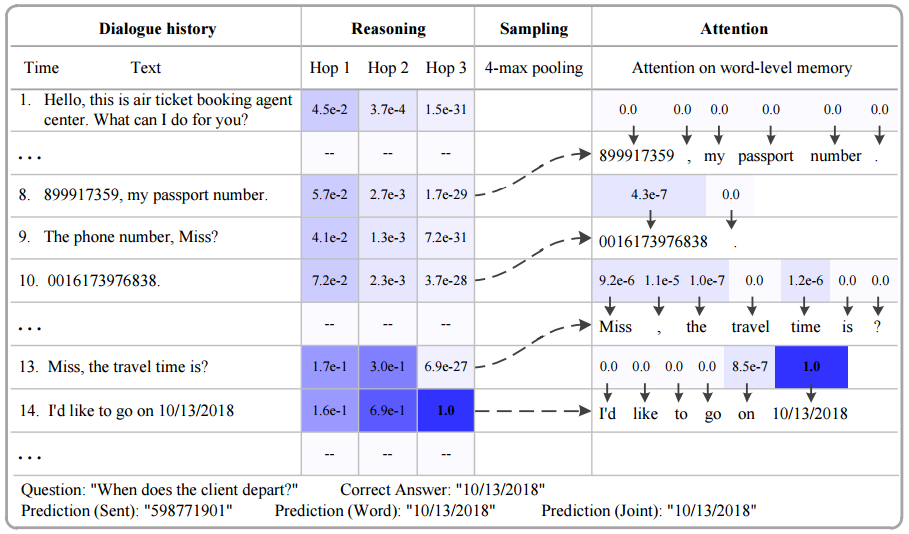# 论文笔记：Hierarchical Memory Networks for Answer Selection on Unknown Words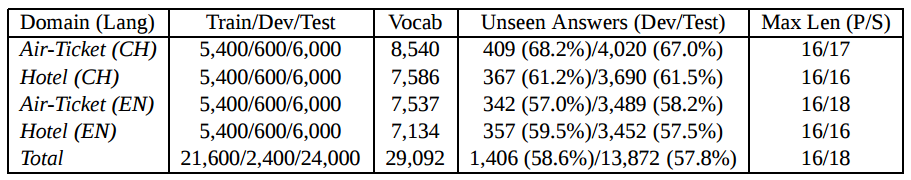1 下午 好 ， 我 是 机票 预订 服务 代理 ， 需要 什么 服务 ？
2 我要 预订 一张 机票 。
3 请问 先生 您 从 哪里 起飞 ？
4 由 BGI 起飞 的 飞机 。
5 去 到 哪里 ？
6 到 印第安纳 去 。
7 电话 号 ？
8 13228762221 ， 这 是 我 的 号码
9 时间 是 ？
10 2015年09月26日22点 之前 。
11 先生 ， 乘客 的 身份证 是 ？
12 我 的 身份证号 是 110100195352319154 。
13 麻烦 说 下 您 的 名字 ？ 谢谢 先生 。
14 好 的 ， 名字 是 袁磊 。
15 先生 ， 我们 已经 成功 为 您 预订 。
16 这么 快 ， 非常 感谢您
17 订票 人 的 姓名 叫 什么 ？ 袁磊 16
18 出发 城市 是 哪里 ？       BGI 16
19 到达 城市 是 哪里 ？       印第安纳 16
20 出发 时间 是 什么 时候 ？  2015年09月26日22点 16
21 证件号码 是 多少 ？        110100195352319154 16
22 联系电话 是 多少 ？        13228762221 16


the memory of these methods, such as Long Short-Term Memory(LSTM) (Hochreiter and Schmidhuber, 1997) and Gated Recurrent Unit (GRU) (Cho et al., 2014) compressing all the external sentences into a fixed-length vector, is typically too small to accurately rememberfacts from the past, and may lose important details for response generation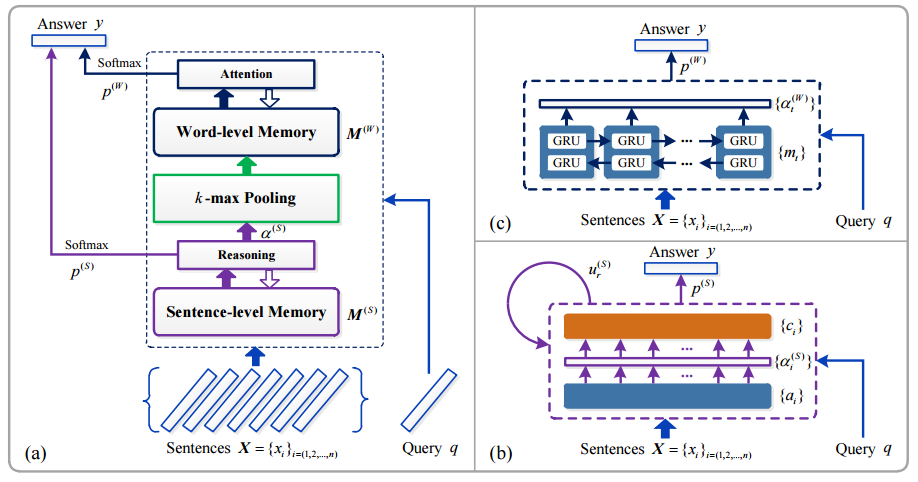1. sentence level memory and reasoning

这部分将 history 信息转换成内部的 memory，并在 此基础上计算结果，同 MemNN，上图中右下块是这部分的图示，要注意的是这里为了简化画成了类似 RNN 的结构图，但并不是说 $$X$$ 中的句子依次输入这个模型，然后更新自连接的 $$u_{r}{(S)}$$，这里的自连接只是表示多层结构(文中使用的层数为 3)，而这种多层结构和 RNN 的结构有共同之处。

在这里，history 也就是 $$X$$ 中的每个句子 $$x_{i}$$ 和 question 也就是 $$q$$ 都要表示成一个向量，使用的是《End-to-End Memory Networks》中的 position encoding 方法，即将句子中每个词的 embedding 加权求和，而这个权值和词在句子中的次序有关。

总之这部分跟《End-to-End Memory Networks》中的内容基本一样，不赘述，详见 MemNN 的笔记

2. k-max pooling

这部分连接 1 和 3，利用 1 输出的 internel state 和 question 一起挑选出 history 中和 question 最相关的 k 个句子。

所谓的 internel state，就是图中的 $$\alpha^{(S)}$$

$\alpha^{(S)}=softmax(M^{T}u_{1}^{(S)})$

上式中 $$M$$ 为 sentence level memory，$$u_{1}$$ 为问题 $$q$$ 的 representation。

3. word level memory and attention: 使用 2 得到的 k 个句子，进行 word level 的 attention 来得到结果

这部分使用 BiGRU 来得到 attention

$M^{(W)} = \{m_{t}\}_{t=(1,2,...)}$

$m_{t}=\overrightarrow{h_{t}}+\overleftarrow{h_{t}}$

$\alpha^{(W)}=softmax(v^{T}\tanh(Wu_{R}^{(S)}+U\hat{m}_{t}))$

这里的 $$alpha^{(W)}$$ 就是 word level 得到的结果，但是这个结果是在 k 个句子中的词上的概率分布，没法直接用于第 4 步的计算，因此要做一次转换，将 $$\alpha^{(W)}$$ 扩充为长度为 $$V$$ 的向量，$$V$$ 是词典的大小，方法是用 0 填充。

4. output: 综合 1 和 3 的输出来计算最后的结果

第一步得到的输出记为 $$p^{(S)}$$，第三步得到的输出记为 $$p^{(W)}$$，将这两者直接相加作为最后的输出

$p = p^{(S)}+p^{(W)}$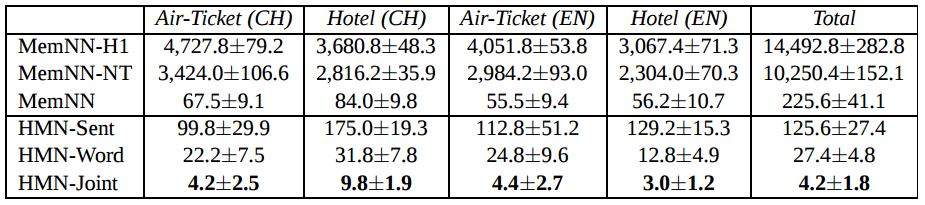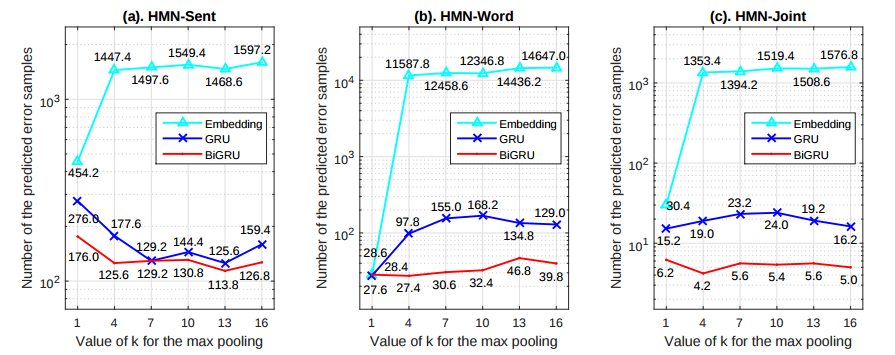1. 表格第一列是 history，这些内容将会被 encode 后储存到 sentence level memory 中
2. 需要回答的问题是: When does the client depart，正确答案是 history 中第 14 条中的日期
3. 表格第二列是 sentense level reasoning 的过程，其中的值是每一个 reasoning 层的 internel state 也就是 $$u_{r}^{(S)}$$，可以看到进行三次 reasoning 后正确的 supporting sentence 也就是第 14 条的权重已经非常的高了
4. 第三列是 k-max pooling，这里的 k 取值为 4，得到的就是 8、10、13、14 这四个候选的句子
5. 第四列是 word level 部分，使用 attention 来得到每个候选句子中的词作为答案的概率，这里概率最大的就是第 14 条句子中的日期